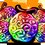I thought of the following problem by myself and would like to post it as a problem; however, I do not know the correct answer to the problem.

Six people choose a whole number from the range 1 to 10 (inclusive) independently and randomly. What is the probability that exactly 2 people will choose the same number and the other 4 people will not choose the same number the 2 people chose and choose numbers different from each other's?

Example: 6, 2, 7, 4, 6, 10 works

Example: 2, 7, 1, 4, 4, 1 doesn't work

Example: 6, 2, 7, 3, 7, 7 doesn't work

I used 2 different strategies to solve the problem and got two different answers of 1260/5005 and 4536/10000. First of all, there can only be one answer to a problem, so at least one of these answers must be wrong, but I checked my strategies and both strategies seem to be flawless. Second, both of the answers seem surprisingly high to me. This isn't a big deal, because sometimes the correct answer to a problem may be surprising, such as the series $1+\frac{1}{4}+\frac{1}{9}...$ being equal to $\frac{\pi^2}{6}$, but even if that is so, I still don't know which of the two answers I got to trust, and I don't even know if either one is correct. If you can solve the problem and reply with the correct answer and why that answer is correct, that would really help. Thanks!

Also, I trust the answer 4536/10000 more than 1260/5005, but I'm not sure enough to post this question as a problem, because 4536/10000 seems very high.Note by Jesse Li
1 week, 6 days ago

MarkdownAppears as
*italics* or _italics_ italics
**bold** or __bold__ bold
- bulleted- list
• bulleted
• list
1. numbered2. list
1. numbered
2. list
Note: you must add a full line of space before and after lists for them to show up correctly
paragraph 1paragraph 2

paragraph 1

paragraph 2

[example link](https://brilliant.org)example link
> This is a quote
This is a quote
    # I indented these lines
# 4 spaces, and now they show
# up as a code block.

print "hello world"
# I indented these lines
# 4 spaces, and now they show
# up as a code block.

print "hello world"
MathAppears as
Remember to wrap math in $ ... $ or $ ... $ to ensure proper formatting.
2 \times 3 $2 \times 3$
2^{34} $2^{34}$
a_{i-1} $a_{i-1}$
\frac{2}{3} $\frac{2}{3}$
\sqrt{2} $\sqrt{2}$
\sum_{i=1}^3 $\sum_{i=1}^3$
\sin \theta $\sin \theta$
\boxed{123} $\boxed{123}$

Sort by:

Hey Jesse. Here's what I got (delightfully!):

The total number of possible choices for the group as a whole is obviously $10^6=1,000,000$ (10 choices per person).

Imagining that the first 4 people will have different guesses and the last 2 have the same, there are $10\times9\times8\times7\times6=30,240$ possible ways for this to happen.

However, there are $5+4+3+2+1=15$ configurations in which this 4/2 split will occur.

Thus we must multiple our original configuration by $15$ to get our total number of acceptable configurations: $453,600$.

To calculate the probability then, we simply divide this number by our total possibilities to get $453,600/1,000,000=\boxed{0.4536}$, the second and more highly trusted of your answers!

Though there may still be some error in any of my assumptions, I was very pleased to have derived an equivalent answer!

If you do post this as a problem, you may want to specify that the configuration has to be exactly 4 non-matching guesses and exactly 2 matching ones for this answer to hold true.

Hope this helped!

- 1 week, 6 days ago

Yeah, this is exactly how my second strategy worked, but I am just unsure of the answer because of how high it is. Thanks for clarifying my answer!

- 1 week, 6 days ago

No problem!

- 1 week, 5 days ago

This is certainly not a proof, but rather a test of the problem:

import random

def run_test(iterations):
print('Running', iterations, 'tests...')
test_results = []

for test in range(iterations):
choices = [random.randint(1, 10) for i in range(6)]
numbers = set(choices)

count_list = []
for number in numbers:
number_count = len([True for choice in choices if choice == number])
count_list.append(number_count)

pair_count = len([count for count in count_list if count == 2])

if pair_count == 1:
test_results.append(True)
else:
test_results.append(False)

return test_results

# Modify the number here \/ to try a greater number of trials
# I wouldn't recommend going too far over 1,000,000 or else the interpreter will run out of execution time :)
test_results = run_test(1000000)

true_count = len([i for i in test_results if i == True])
false_count = len([i for i in test_results if i == False])
total = true_count + false_count

probability = true_count/total

print('Permissable:', true_count)
print('Not permissable:', false_count)
print('The probability then of obtaining a permissable set is:', probability)


- 1 week, 2 days ago